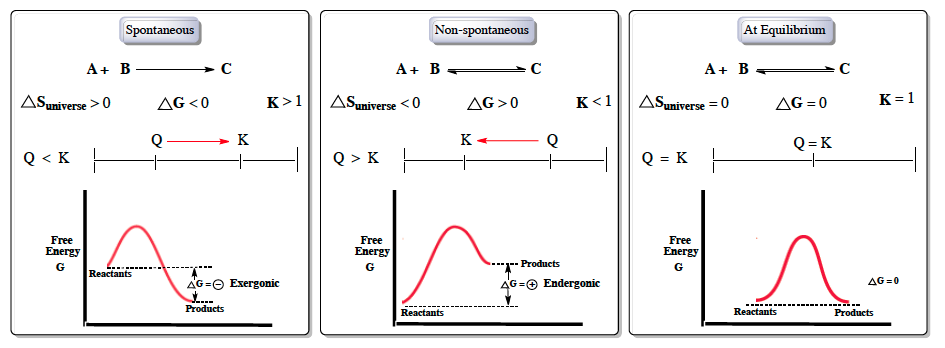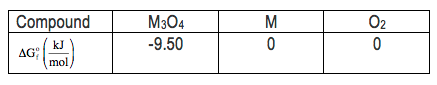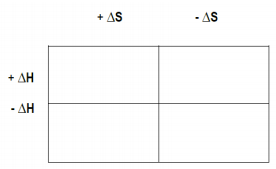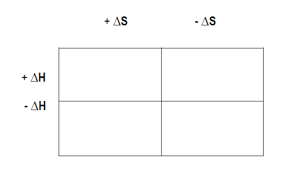Ch.6 - Chemical EquilibriumWorksheetSee all chapters
 Ch.1 - Chemical Measurements 2hrs & 8mins 0% complete WorksheetDownload the video lesson worksheet Ch.2 - Tools of the Trade 1hr & 26mins 0% complete WorksheetDownload the video lesson worksheet Ch.3 - Experimental Error 1hr & 53mins 0% complete WorksheetDownload the video lesson worksheet Ch.4 + 5 - Statistics, Quality Assurance and Calibration Methods 2hrs & 1min 0% complete WorksheetDownload the video lesson worksheet Ch.6 - Chemical Equilibrium 3hrs & 43mins 0% complete WorksheetDownload the video lesson worksheet Ch.7 - Activity and the Systematic Treatment of Equilibrium 1hr & 1min 0% complete WorksheetDownload the video lesson worksheet Ch.8 - Monoprotic Acid-Base Equilibria 1hr & 52mins 0% complete WorksheetDownload the video lesson worksheet Ch.9 - Polyprotic Acid-Base Equilibria 2hrs & 16mins 0% complete WorksheetDownload the video lesson worksheet Ch.10 - Acid-Base Titrations 2hrs & 40mins 0% complete WorksheetDownload the video lesson worksheet Ch.11 - EDTA Titrations 1hr & 37mins 0% complete WorksheetDownload the video lesson worksheet Ch.12 - Advanced Topics in Equilibrium 1hr & 18mins 0% complete WorksheetDownload the video lesson worksheet Ch.13 - Fundamentals of Electrochemistry 2hrs & 27mins 0% complete WorksheetDownload the video lesson worksheet Ch.14 - Electrodes and Potentiometry 43mins 0% complete WorksheetDownload the video lesson worksheet Ch.15 - Redox Titrations 1hr & 14mins 0% complete WorksheetDownload the video lesson worksheet Ch.16 - Electroanalytical Techniques 1hr & 3mins 0% complete WorksheetDownload the video lesson worksheet Ch.17 - Fundamentals of Spectrophotometry 55mins 0% complete WorksheetDownload the video lesson worksheet BONUS: Chemical Kinetics Not available yet

# Chemical Thermodynamics: Gibbs Free Energy

See all sections
Sections
The Equilibrium State
The Reaction Quotient
Le Chatelier's Principle
Chemical Thermodynamics: Enthalpy
Chemical Thermodynamics: Entropy
Chemical Thermodynamics: Gibbs Free Energy
Solubilty Product Constant
Protic Acids and Bases
The pH Scale
Acid Strength

Gibbs Free Energy represents the maximum amount of work that can be done by a thermodynamic reaction at constant pressure and temperature.

###### Understanding Gibbs Free Energy

Concept #1: The signs of Gibbs Free Energy can be used to determine if a reaction is spontaneous or non-spontaneous.Example #1: Which of the following is an example of a nonspontaneous process?

a) Ice melting at room temperature

b) Sodium metal reacting violently with water

c) Rusting of iron at room temperature

d) A ball rolling downhill

e) Water freezing at room temperature

###### Gibbs Free Energy Calculations

Example #2: Consider the decomposition of a metal oxide to its elements, where M represents a generic metal.

M3O4 (s) ⇌ 3 M (s) + 2 O(g)

i) What is the standard change in Gibbs energy for the reaction, as written, in the forward direction?

ii) What is the equilibrium constant of this reaction, as written, in the forward direction at 298 K?

iii) What is the equilibrium pressure of 0(g) over M (s) at 298 K?Example #3: For the reaction:

2 C (graphite) + H2 (g) → C2H2 (g)

ΔG° = + 209.2 kJ at 25°C. If PH2 = 100 atm, and PC2H2 = 0.10 atm, calculate ΔG for reaction.

a) +192.1 kJ
b) +266.3 kJ
c) -16.9 kJ
d) +207.8 kJ
e) +17.3 kJ

Example #4: Sodium carbonate can be made by heating sodium bicarbonate:

2 NaHCO3 (s) →  Na2CO3 (s) + CO2 (g) + H2O (g)

Given that ΔH° = 128.9 kJ/mol and ΔG° = 33.1 kJ/mol at 25°C, above what minimum temperature will the reaction become spontaneous under standard-state conditions?

a) 0.4 K

B) 3.9 K

C) 321 K

D) 401 K

E) 525 K

Practice: The signs of ∆H, ∆S, and ∆G at 25oC are shown below for three chemical reactions.

∆H      ∆S     ∆GWhich reaction would go in the reverse direction at high temperatures?